See The Largest Known Prime, All 13 Million Digits The largest prime number ever found weighs in at 13 million digits long. There are only 46 of these rare, huge numbers -- so far -- and mathematicians are continuing the search by using the computing power of the Internet. Just what makes these numbers so special?

See The Largest Known Prime, All 13 Million Digits

In Depth

We all think we remember prime numbers from grade school, but just in case your memory is a little hazy, here's a quick refresher.

A prime is a number that can only be divided by two other whole numbers: itself and 1.

So, 1? Not a prime -- it can only be divided by 1. You need two divisors to qualify for prime status.

Marked in orange are some of the easy primes -- because they don't take much brain power to figure out: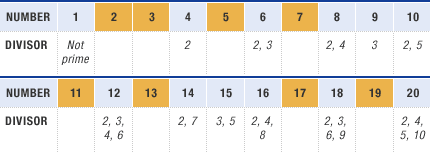But then, there are the special primes. These numbers come from a variety of simple equations, but present mind-bogglingly large numbers. Finding primes was sort of an elite hobby for some of the early math greats, like Pierre de Fermat, Carl Gauss and Sophie Germain.

But the most celebrated of all primes is the Mersenne -- what mathematician Chris Caldwell calls "the jewels of number theory."

Rare, Huge Numbers

Mersenne primes, named after 17th-century mathematician Marin Mersenne, are numbers derived from the simple equation: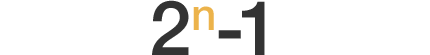The number n is a prime, and the result is prime. And what makes the Mersenne primes so interesting is how rare they are. And their gargantuan size.

Currently, a Mersenne prime clocking in at nearly 13 million digits holds the record of being the largest prime number ever found. You can take a look at just a snippet of the number here. But if you want to see all 13 million digits, you can download this whopping 17-megabyte text file. (That's almost 3,500 single-spaced pages in a Word document, at 12-point Times New Roman font.)

And here's a glimpse at how quickly Mersennes ramp up in size: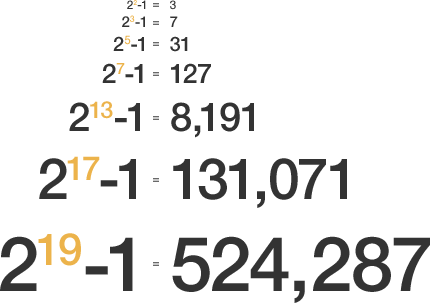Notice that as the value of the number n -- the exponents 2, 3, 5, 7, 13, 17 and 19 in the example above -- increases, the value of the Mersenne prime skyrockets. If we plot the value of the Mersenne prime vs. the value of the exponent, here's what the pattern looks like: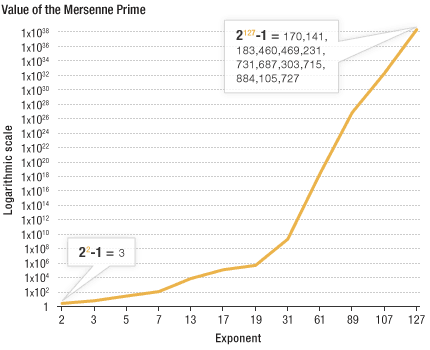And here's an easier way to think of that Mersenne prime at the top right of the chart: It's more than a trillion trillion trillion. You get that number with this equation: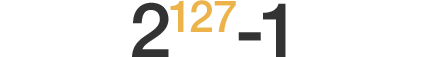So just using an exponent of 127 gives you that huge number. But the largest Mersenne prime's exponent is 43,112,609.

To find such a large number is beyond the brain power of anyone. Microsoft Excel can't even calculate any Mersenne prime with an exponent larger than 607. If you try, it gives you a nice #NUM! error -- that's Excelese for FAIL.

You need massive computers to do the number crunching. So a team called the Great Internet Mersenne Prime Search, or GIMPS, is farming the computing power out to the Internet. Of the 46 Mersenne primes found so far, GIMPS has discovered 12.

Users can lend their desktop computing power out to a program using a type of software called distributed computing. This type of large-scale marshaling of computing power is not new to the Internet. Other groups, like the Search For Extraterrestrial Intelligence, or SETI@home, use personal computers for data crunching, as does the Folding@home program, based at Stanford University, whose goal is to learn more about how the proteins found in living organisms fold into complex shapes and interact with other biological molecules.

So why bother searching for these primes? Any math geek will tell you that it's just cool and they're in it for the chase. But the effort has been reciprocal, too. As they search for these ever-larger primes, they have also been able to contribute important theorems and principles to the field of mathematics.